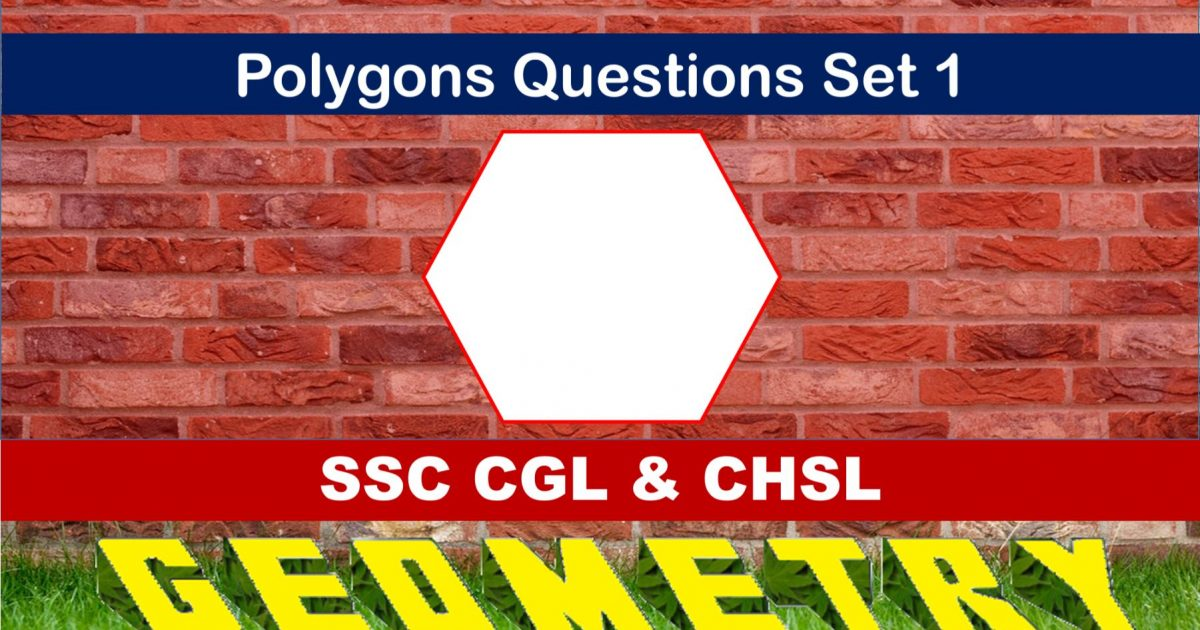Monday, September 27, 2021
Home > geometry > SSC CGL Geometry Polygon Set 1

# SSC CGL Geometry Polygon Set 1SSC CGL Geometry Polygon Set 1 : Ques No 1

The ratio between the number of sides of two regular polygons is 1 : 2 and the ratio between their interior angles are 2 : 3. The number of sides of the polygons are respectively

Options:

A. 5. 10

B. 6, 12

C. 7, 14

D. 4, 8

Solution:

SSC CGL Geometry Polygon Set 1 : Ques No 2

If the ratio of an external angle and an internal angle of a regular polygon is 1 : 17, the number of sides of the regular polygon is

Options:

A. 12

B. 36

C. 20

D. 18

Solution:

SSC CGL Geometry Polygon Set 1 : Ques No 3

If the ratio number of sides of two polygons is 5 : 6 and the ratio of their internal angles is 24 : 25, the number of sides of two polygons are

Options:

A. 20, 24

B. 5, 6

C. 15, 18

D. 10, 12

Solution:

SSC CGL Geometry Polygon Set 1 : Ques No 4

An interior angle of a regular polygon is five times its exterior angle. Then the number of sides of the polygon is

Options:

A. 16

B. 18

C. 12

D. 14

Solution:

SSC CGL Geometry Polygon Set 1 : Ques No 5

One angle of a pentagon is 140 degree. If the remaining angles are in the ratio 1 : 2 : 3 : 4, then find the  value of greatest angle.

Options:

A. 150 degree

B. 180 degree

C. 160 degree

D. 170 degree

Solution:

SSC CGL Geometry Polygon Set 1 : Ques No 6

The sum of all exterior angles of a convex polygon of n sides is

Options:

A. 4 Right Angles

B. 2/n Right Angles

C. (2n – 4) Right Angles

D. n/2 Right Angles

Solution:SSC CGL Geometry Polygon Set 1 : Ques No 7

The sum of the interior angles of polygon is 1440 degrees. The number of sides of the polygon is

Options:

A. 8

B. 9

C. 10

D. 12

Solution:

SSC CGL Geometry Polygon Set 1 : Ques No 8

The ratio of measure of an interior angle of a regular octagon to the measure of its exterior angle is

Options:

A. 1 : 3

B. 2 : 3

C. 3 : 1

D. 3 : 2

Solution:

SSC CGL Geometry Polygon Set 1 : Ques No 9

The sum of all the interior angles of regular polygon is four times the sum of its exterior angles. The polygon is

Options:

A. Hexagon

B. Triangle

C. Decagon

D. Nonagon

Solution:

SSC CGL Geometry Polygon Set 1 : Ques No 10

A polygon has 54 diagonals. The number of sides in the polygon is

Options:

A. 7

B. 9

C. 12

D. 19

Solution:

SSC CGL Geometry Polygon Set 1 : Ques No 11

If each interior angle of a regular polygon is 3 times its exterior angle, the number of sides of the polygon is

Options:

A. 4

B. 5

C. 6

D. 8

Solution:

SSC CGL Geometry Polygon Set 1 : Ques No 12

The number of diagonals of a polygon having 25 sides is

Options:

A. 250

B. 240

C. 275

D. 265

Solution:

SSC CGL Geometry Polygon Set 1 : Ques No 13

A polygon has 20 sides. If one of its vertices of the polygon does not send any diagonal, then the total number of diagonals in the polygon is

Options:

A. 170

B. 153

C. 160

D. 147

Solution:

SSC CGL Geometry Polygon Set 1 : Ques No 14

A polygon has 18 sides. If three of its vertices of the polygon do not send any diagonal, then the total number of diagonals in the polygon is

Options:

A. 56

B. 90

C. 120

D. 135